Скачать презентацию C 142 B Campbell Callis Chapter 2

a258397e505e894df534bbbf1a525436.ppt

• Количество слайдов: 40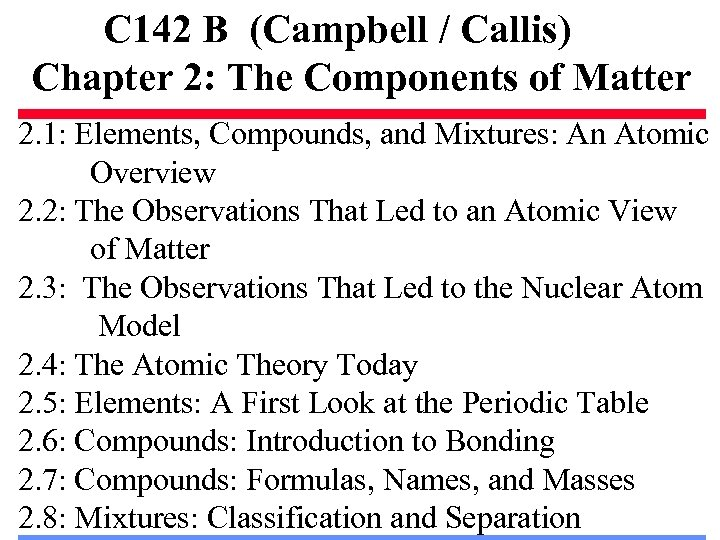C 142 B (Campbell / Callis) Chapter 2: The Components of Matter 2. 1: Elements, Compounds, and Mixtures: An Atomic Overview 2. 2: The Observations That Led to an Atomic View of Matter 2. 3: The Observations That Led to the Nuclear Atom Model 2. 4: The Atomic Theory Today 2. 5: Elements: A First Look at the Periodic Table 2. 6: Compounds: Introduction to Bonding 2. 7: Compounds: Formulas, Names, and Masses 2. 8: Mixtures: Classification and Separation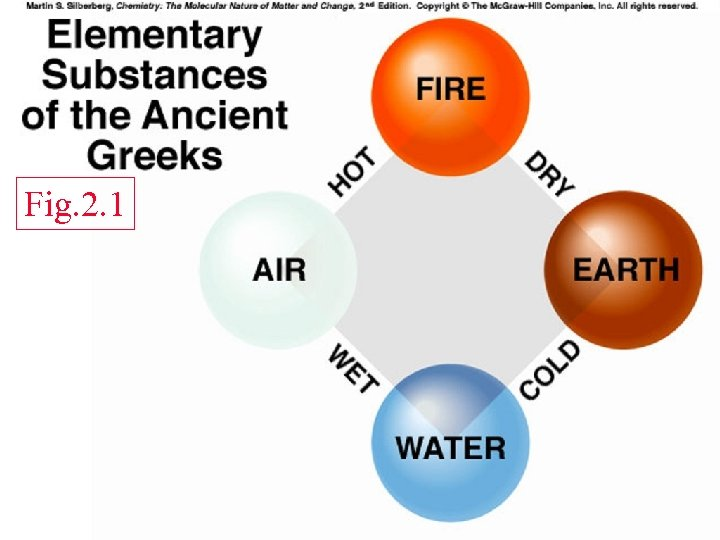Fig. 2. 1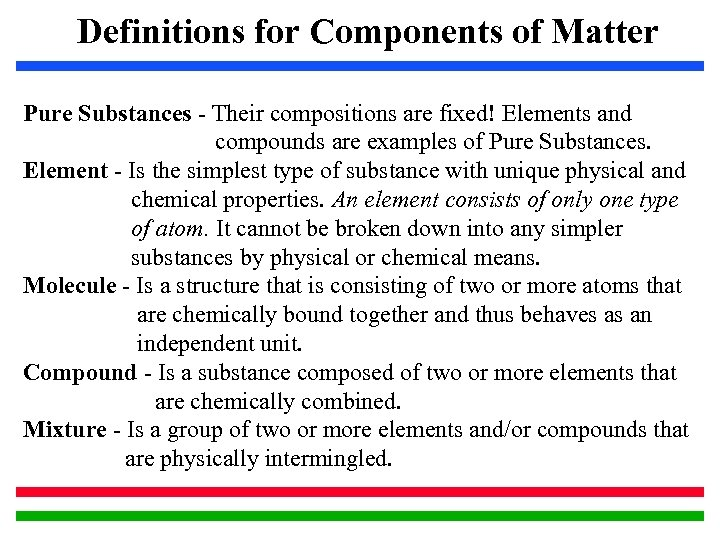Definitions for Components of Matter Pure Substances - Their compositions are fixed! Elements and compounds are examples of Pure Substances. Element - Is the simplest type of substance with unique physical and chemical properties. An element consists of only one type of atom. It cannot be broken down into any simpler substances by physical or chemical means. Molecule - Is a structure that is consisting of two or more atoms that are chemically bound together and thus behaves as an independent unit. Compound - Is a substance composed of two or more elements that are chemically combined. Mixture - Is a group of two or more elements and/or compounds that are physically intermingled.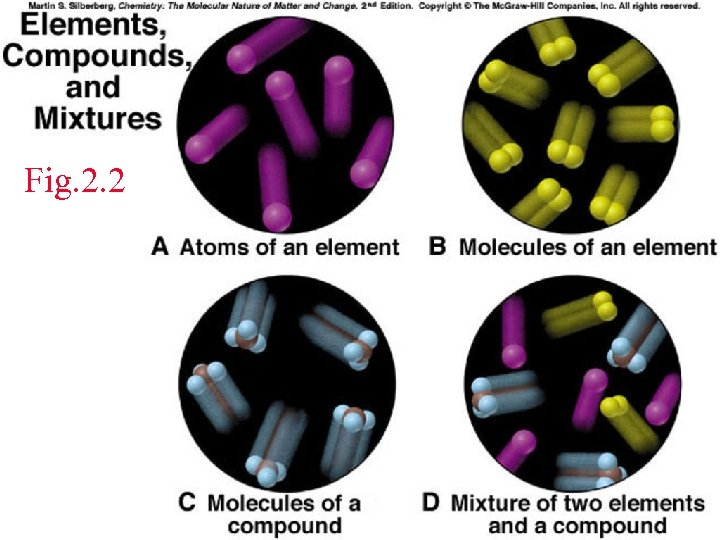Fig. 2. 2Fig 2. 4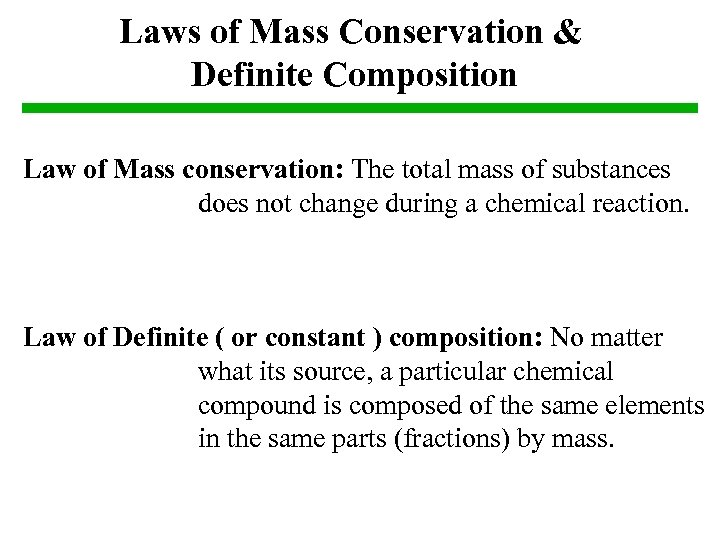Laws of Mass Conservation & Definite Composition Law of Mass conservation: The total mass of substances does not change during a chemical reaction. Law of Definite ( or constant ) composition: No matter what its source, a particular chemical compound is composed of the same elements in the same parts (fractions) by mass.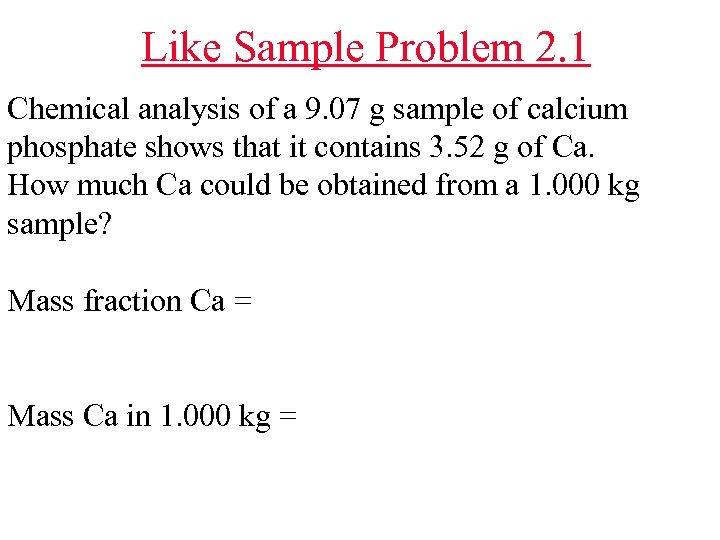Like Sample Problem 2. 1 Chemical analysis of a 9. 07 g sample of calcium phosphate shows that it contains 3. 52 g of Ca. How much Ca could be obtained from a 1. 000 kg sample? Mass fraction Ca = Mass Ca in 1. 000 kg =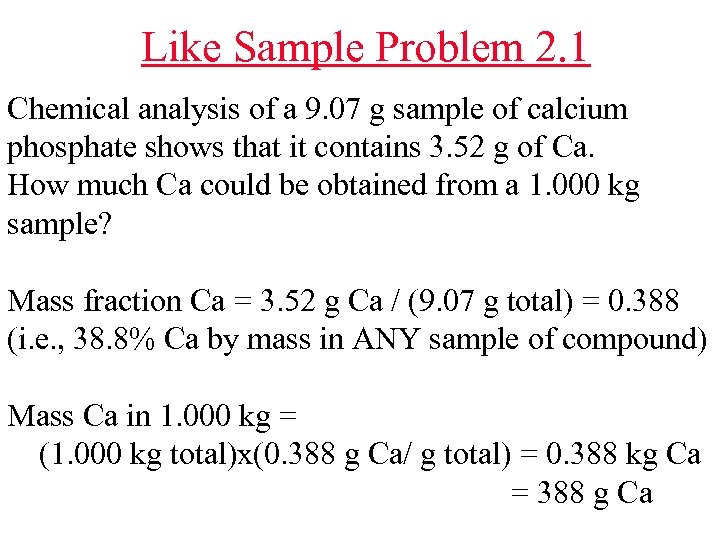Like Sample Problem 2. 1 Chemical analysis of a 9. 07 g sample of calcium phosphate shows that it contains 3. 52 g of Ca. How much Ca could be obtained from a 1. 000 kg sample? Mass fraction Ca = 3. 52 g Ca / (9. 07 g total) = 0. 388 (i. e. , 38. 8% Ca by mass in ANY sample of compound) Mass Ca in 1. 000 kg = (1. 000 kg total)x(0. 388 g Ca/ g total) = 0. 388 kg Ca = 388 g CaFig. 2. 5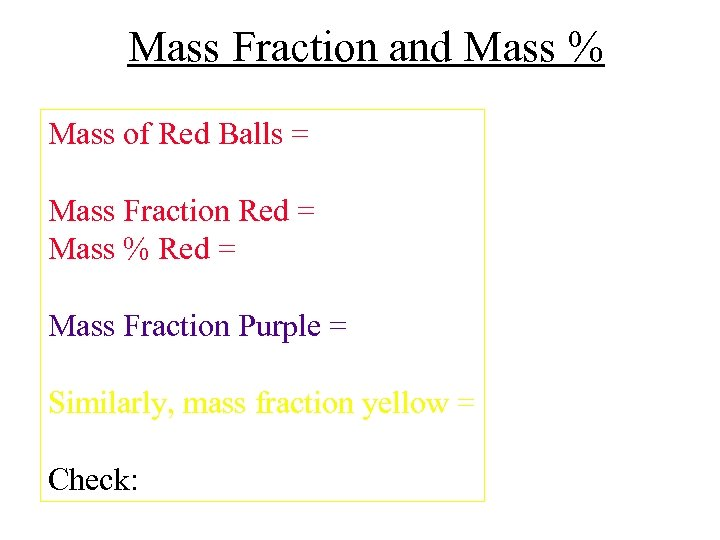Mass Fraction and Mass % Mass of Red Balls = Mass Fraction Red = Mass % Red = Mass Fraction Purple = Similarly, mass fraction yellow = Check: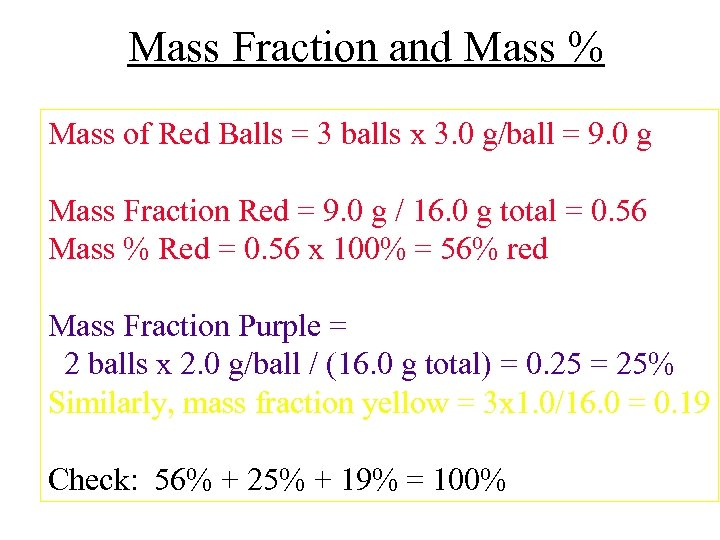Mass Fraction and Mass % Mass of Red Balls = 3 balls x 3. 0 g/ball = 9. 0 g Mass Fraction Red = 9. 0 g / 16. 0 g total = 0. 56 Mass % Red = 0. 56 x 100% = 56% red Mass Fraction Purple = 2 balls x 2. 0 g/ball / (16. 0 g total) = 0. 25 = 25% Similarly, mass fraction yellow = 3 x 1. 0/16. 0 = 0. 19 Check: 56% + 25% + 19% = 100%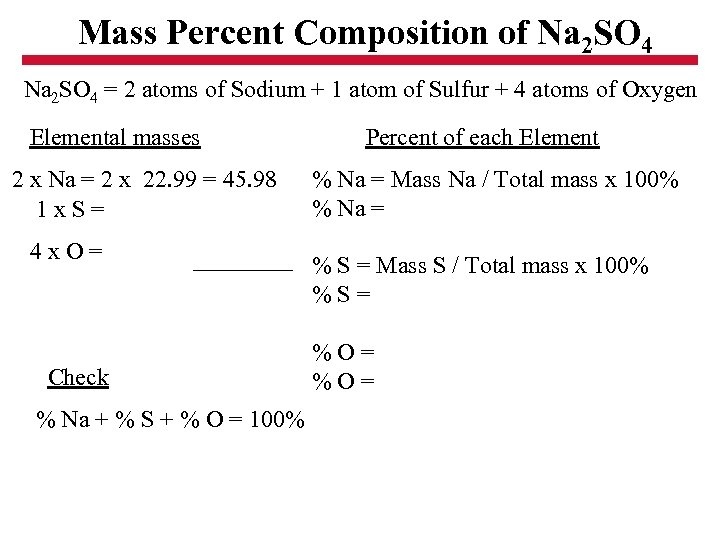Mass Percent Composition of Na 2 SO 4 = 2 atoms of Sodium + 1 atom of Sulfur + 4 atoms of Oxygen Elemental masses 2 x Na = 2 x 22. 99 = 45. 98 1 x. S= 4 x. O= Check % Na + % S + % O = 100% Percent of each Element % Na = Mass Na / Total mass x 100% % Na = % S = Mass S / Total mass x 100% %S= %O=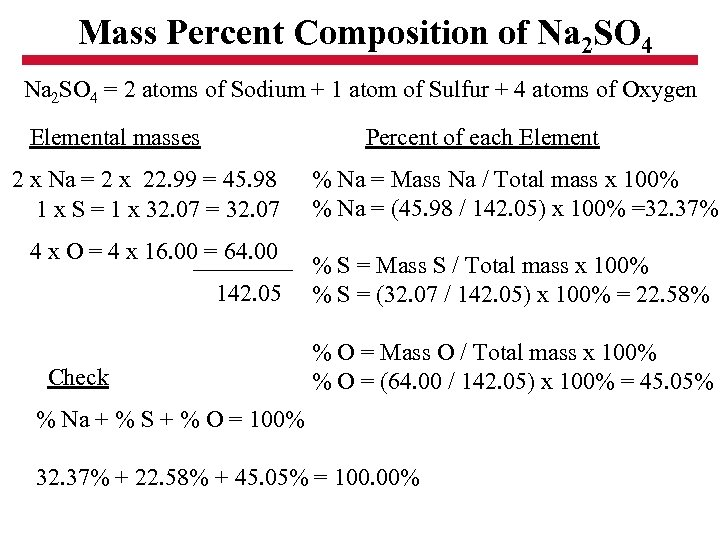Mass Percent Composition of Na 2 SO 4 = 2 atoms of Sodium + 1 atom of Sulfur + 4 atoms of Oxygen Elemental masses Percent of each Element 2 x Na = 2 x 22. 99 = 45. 98 1 x S = 1 x 32. 07 = 32. 07 4 x O = 4 x 16. 00 = 64. 00 142. 05 Check % Na = Mass Na / Total mass x 100% % Na = (45. 98 / 142. 05) x 100% =32. 37% % S = Mass S / Total mass x 100% % S = (32. 07 / 142. 05) x 100% = 22. 58% % O = Mass O / Total mass x 100% % O = (64. 00 / 142. 05) x 100% = 45. 05% % Na + % S + % O = 100% 32. 37% + 22. 58% + 45. 05% = 100. 00%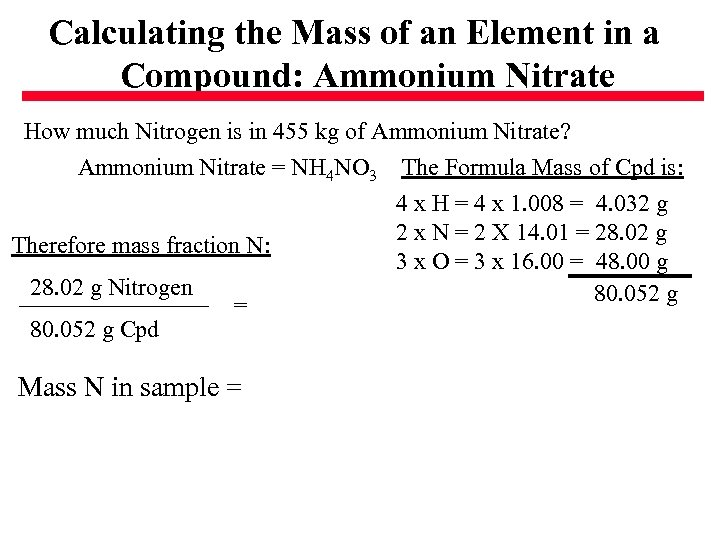Calculating the Mass of an Element in a Compound: Ammonium Nitrate How much Nitrogen is in 455 kg of Ammonium Nitrate? Ammonium Nitrate = NH 4 NO 3 Therefore mass fraction N: 28. 02 g Nitrogen 80. 052 g Cpd = Mass N in sample = The Formula Mass of Cpd is: 4 x H = 4 x 1. 008 = 4. 032 g 2 x N = 2 X 14. 01 = 28. 02 g 3 x O = 3 x 16. 00 = 48. 00 g 80. 052 g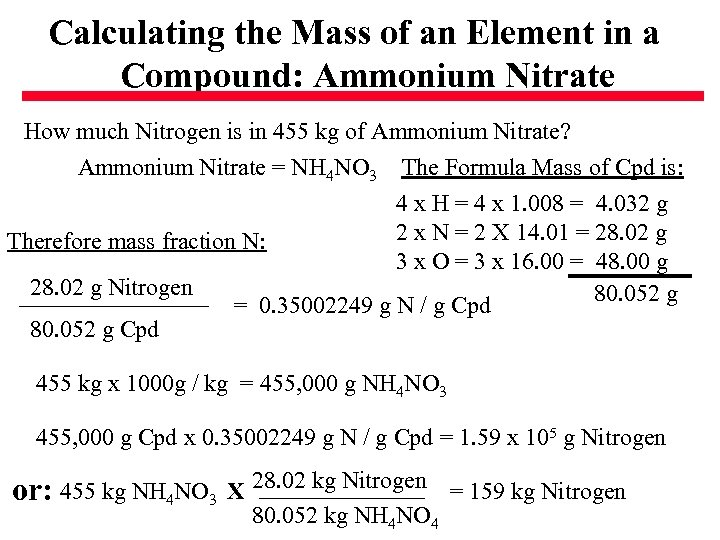Calculating the Mass of an Element in a Compound: Ammonium Nitrate How much Nitrogen is in 455 kg of Ammonium Nitrate? Ammonium Nitrate = NH 4 NO 3 The Formula Mass of Cpd is: 4 x H = 4 x 1. 008 = 4. 032 g 2 x N = 2 X 14. 01 = 28. 02 g Therefore mass fraction N: 3 x O = 3 x 16. 00 = 48. 00 g 28. 02 g Nitrogen 80. 052 g = 0. 35002249 g N / g Cpd 80. 052 g Cpd 455 kg x 1000 g / kg = 455, 000 g NH 4 NO 3 455, 000 g Cpd x 0. 35002249 g N / g Cpd = 1. 59 x 105 g Nitrogen or: 455 kg NH 4 NO 3 X 28. 02 kg Nitrogen = 159 kg Nitrogen 80. 052 kg NH 4 NO 4Fig. 2. 7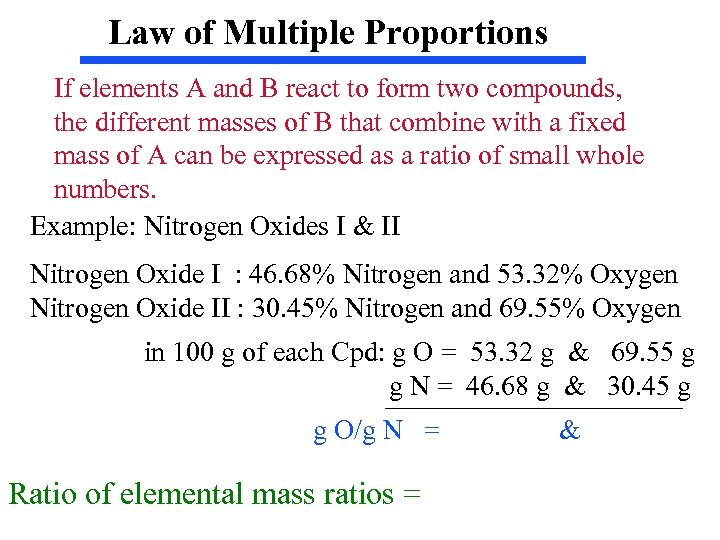Law of Multiple Proportions If elements A and B react to form two compounds, the different masses of B that combine with a fixed mass of A can be expressed as a ratio of small whole numbers. Example: Nitrogen Oxides I & II Nitrogen Oxide I : 46. 68% Nitrogen and 53. 32% Oxygen Nitrogen Oxide II : 30. 45% Nitrogen and 69. 55% Oxygen in 100 g of each Cpd: g O = 53. 32 g & 69. 55 g g N = 46. 68 g & 30. 45 g g O/g N = Ratio of elemental mass ratios = &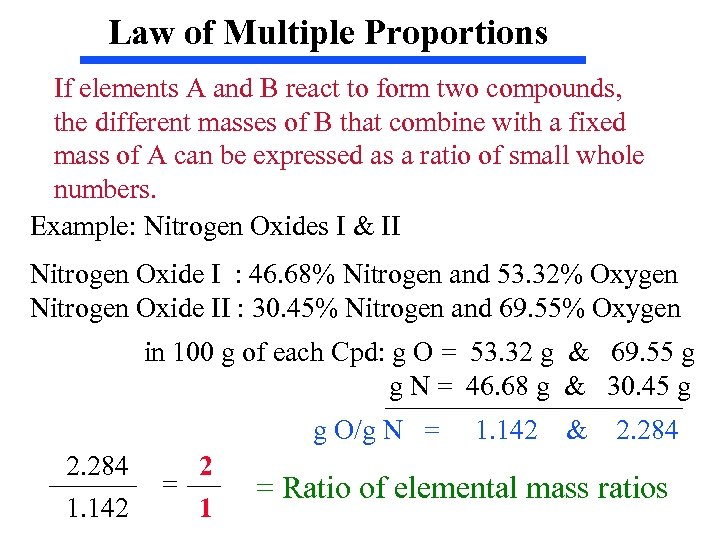Law of Multiple Proportions If elements A and B react to form two compounds, the different masses of B that combine with a fixed mass of A can be expressed as a ratio of small whole numbers. Example: Nitrogen Oxides I & II Nitrogen Oxide I : 46. 68% Nitrogen and 53. 32% Oxygen Nitrogen Oxide II : 30. 45% Nitrogen and 69. 55% Oxygen in 100 g of each Cpd: g O = 53. 32 g & 69. 55 g g N = 46. 68 g & 30. 45 g g O/g N = 2. 284 1. 142 2 = 1 1. 142 & 2. 284 = Ratio of elemental mass ratios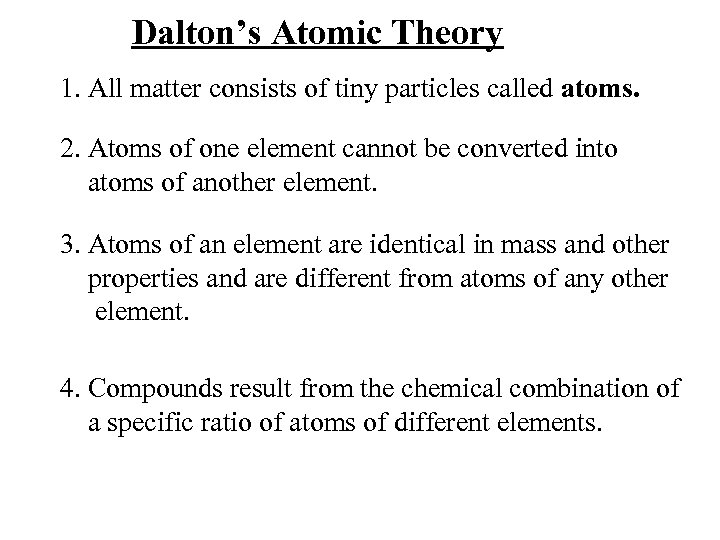Dalton’s Atomic Theory 1. All matter consists of tiny particles called atoms. 2. Atoms of one element cannot be converted into atoms of another element. 3. Atoms of an element are identical in mass and other properties and are different from atoms of any other element. 4. Compounds result from the chemical combination of a specific ratio of atoms of different elements.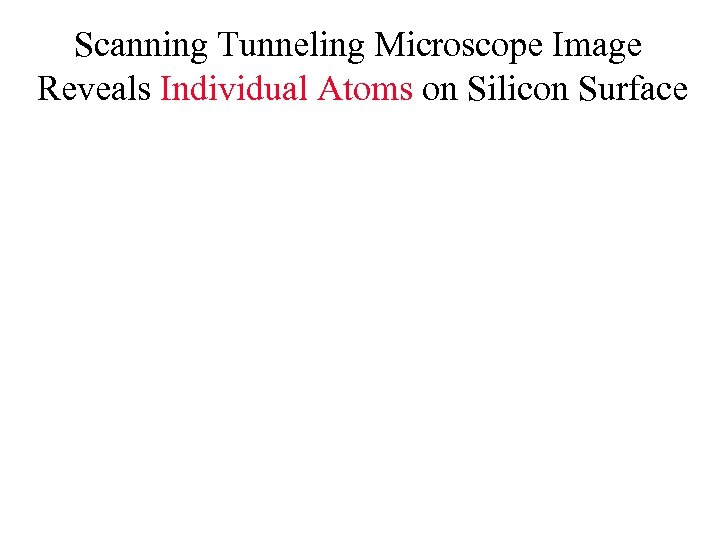Scanning Tunneling Microscope Image Reveals Individual Atoms on Silicon Surface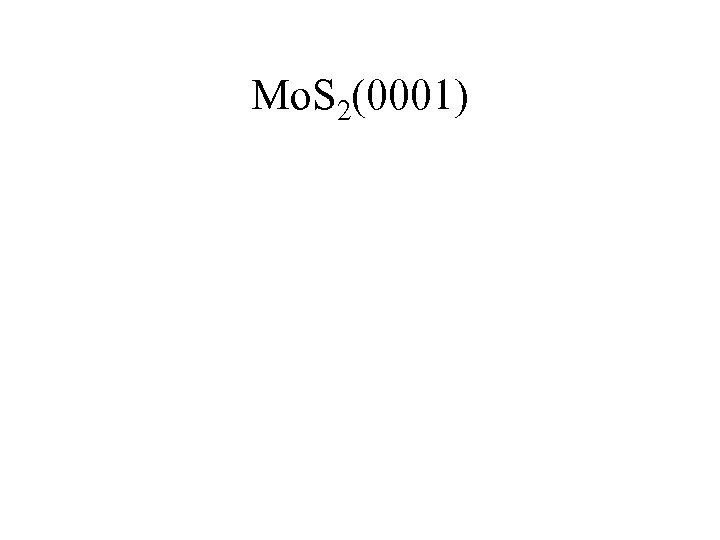Mo. S 2(0001)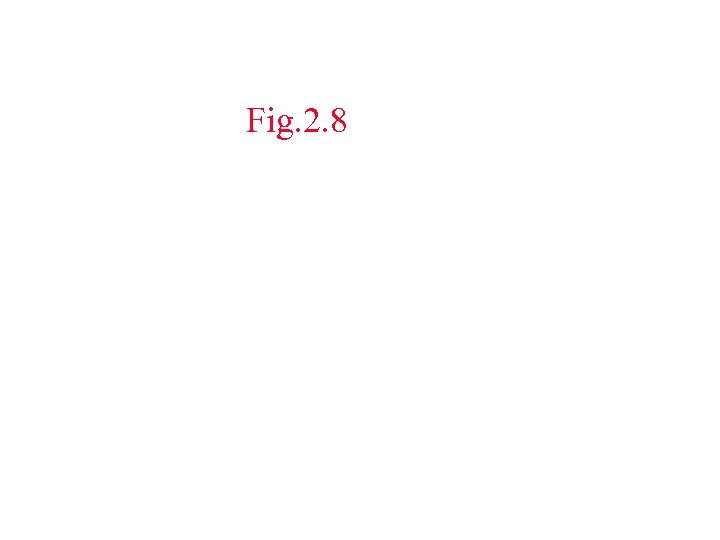Fig. 2. 8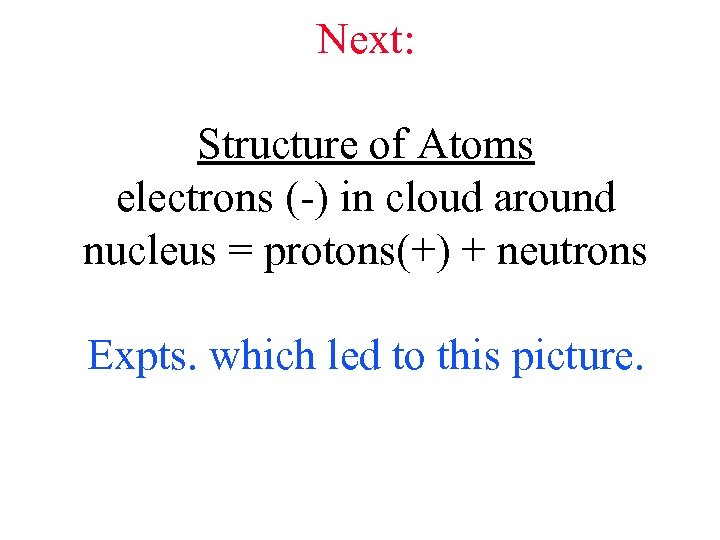Next: Structure of Atoms electrons (-) in cloud around nucleus = protons(+) + neutrons Expts. which led to this picture.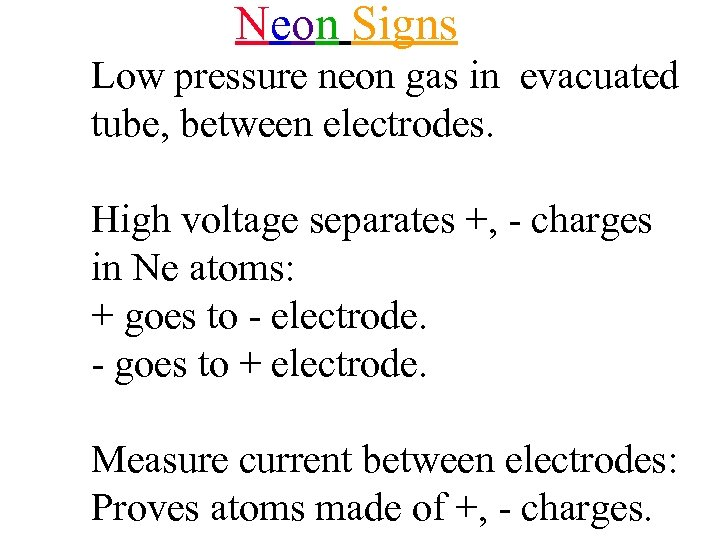Neon Signs Low pressure neon gas in evacuated tube, between electrodes. High voltage separates +, - charges in Ne atoms: + goes to - electrode. - goes to + electrode. Measure current between electrodes: Proves atoms made of +, - charges.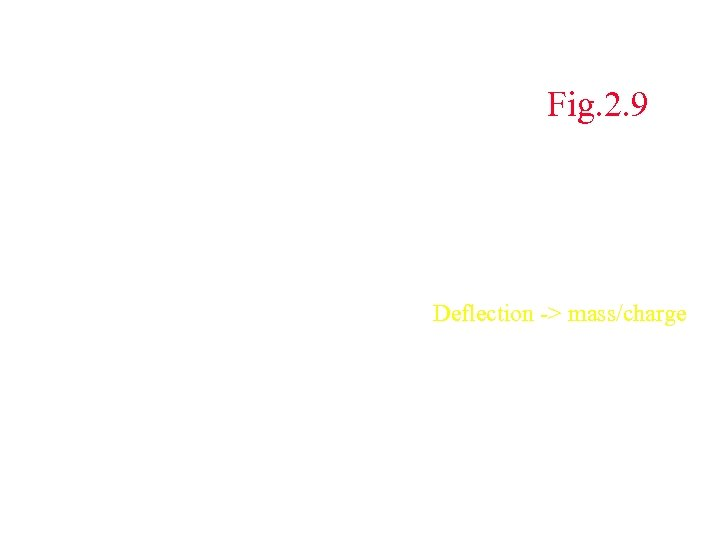Fig. 2. 9 Deflection -> mass/charge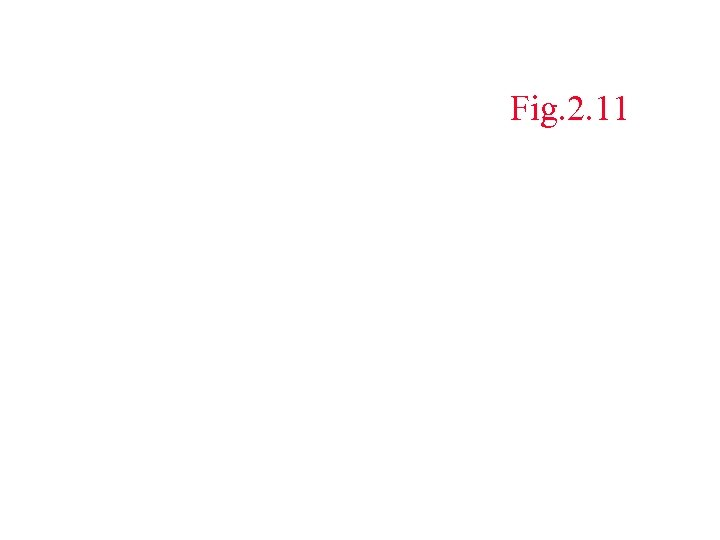Fig. 2. 11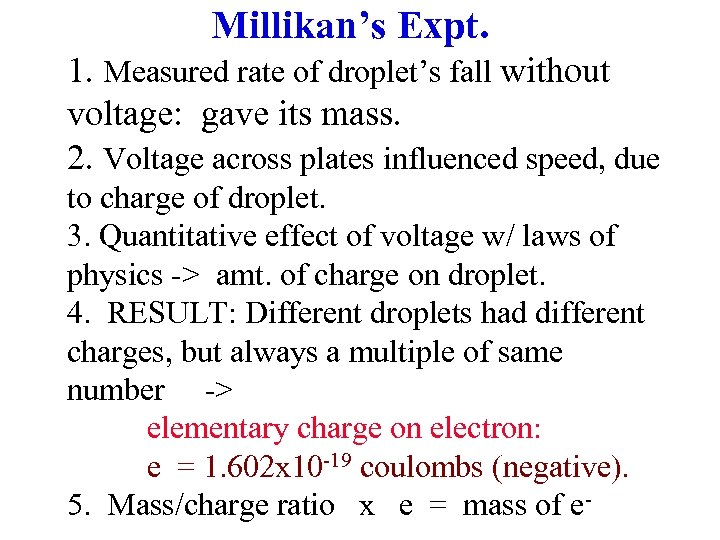Millikan’s Expt. 1. Measured rate of droplet’s fall without voltage: gave its mass. 2. Voltage across plates influenced speed, due to charge of droplet. 3. Quantitative effect of voltage w/ laws of physics -> amt. of charge on droplet. 4. RESULT: Different droplets had different charges, but always a multiple of same number -> elementary charge on electron: e = 1. 602 x 10 -19 coulombs (negative). 5. Mass/charge ratio x e = mass of e-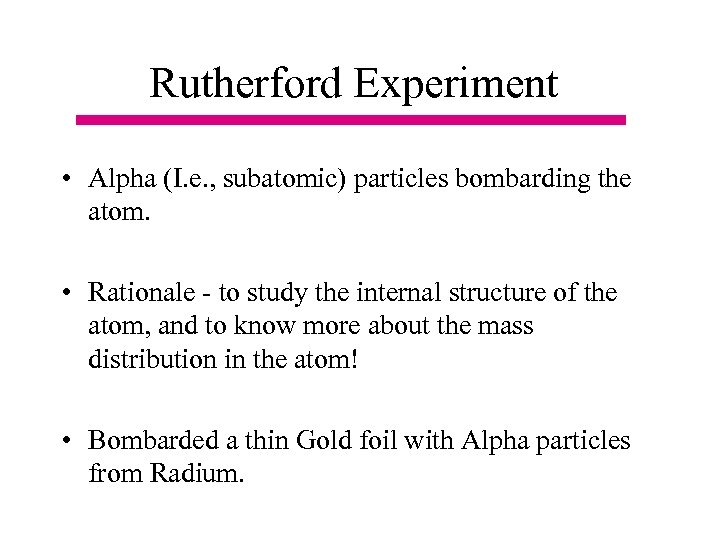Rutherford Experiment • Alpha (I. e. , subatomic) particles bombarding the atom. • Rationale - to study the internal structure of the atom, and to know more about the mass distribution in the atom! • Bombarded a thin Gold foil with Alpha particles from Radium.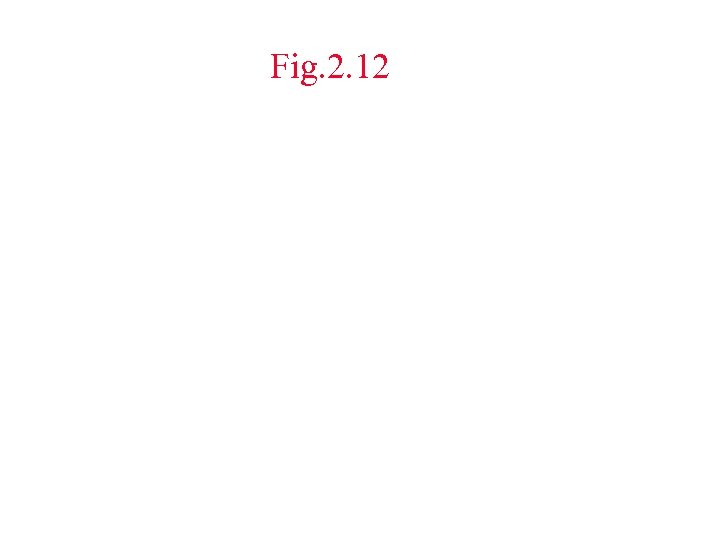Fig. 2. 12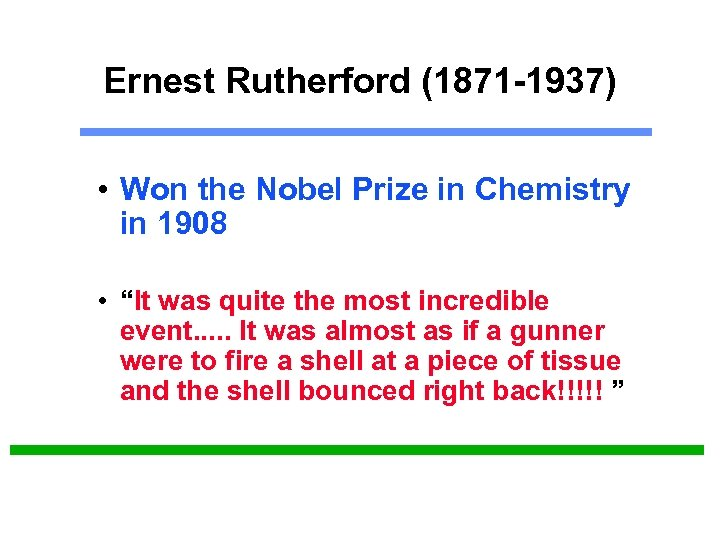Ernest Rutherford (1871 -1937) • Won the Nobel Prize in Chemistry in 1908 • “It was quite the most incredible event. . . It was almost as if a gunner were to fire a shell at a piece of tissue and the shell bounced right back!!!!! ”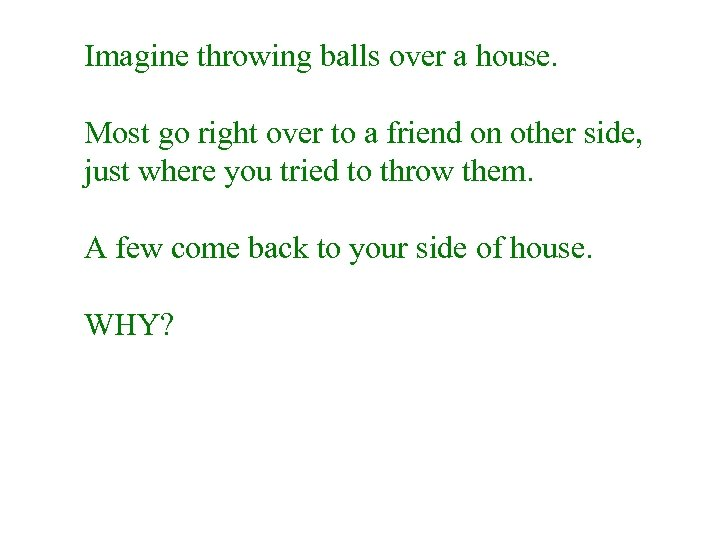Imagine throwing balls over a house. Most go right over to a friend on other side, just where you tried to throw them. A few come back to your side of house. WHY?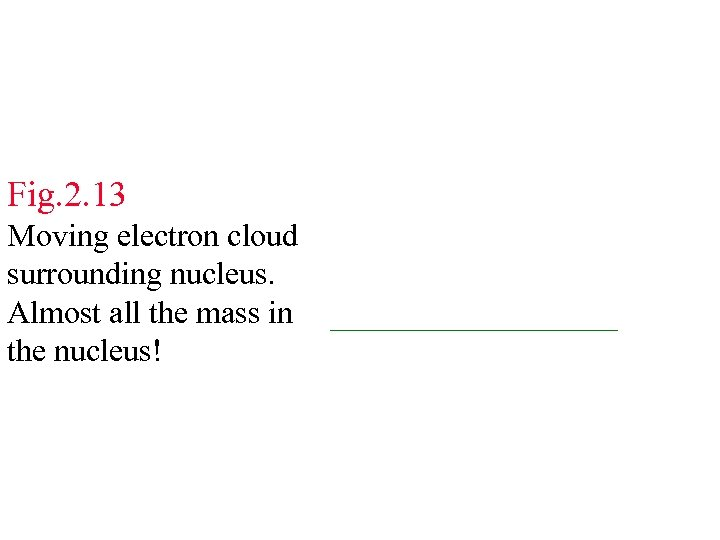Fig. 2. 13 Moving electron cloud surrounding nucleus. Almost all the mass in _________ the nucleus!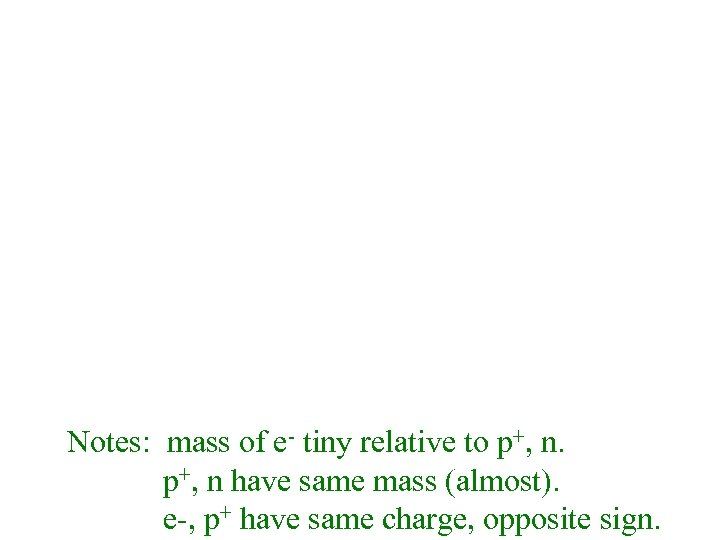Notes: mass of e- tiny relative to p+, n have same mass (almost). e-, p+ have same charge, opposite sign.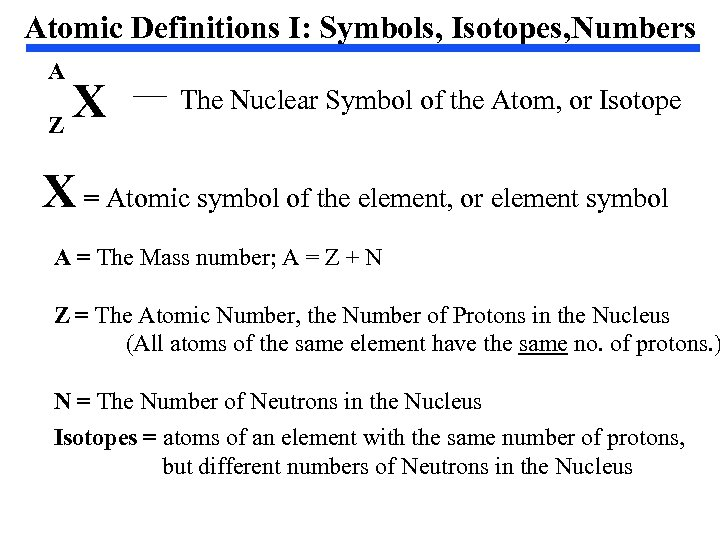Atomic Definitions I: Symbols, Isotopes, Numbers A X Z The Nuclear Symbol of the Atom, or Isotope X = Atomic symbol of the element, or element symbol A = The Mass number; A = Z + N Z = The Atomic Number, the Number of Protons in the Nucleus (All atoms of the same element have the same no. of protons. ) N = The Number of Neutrons in the Nucleus Isotopes = atoms of an element with the same number of protons, but different numbers of Neutrons in the Nucleus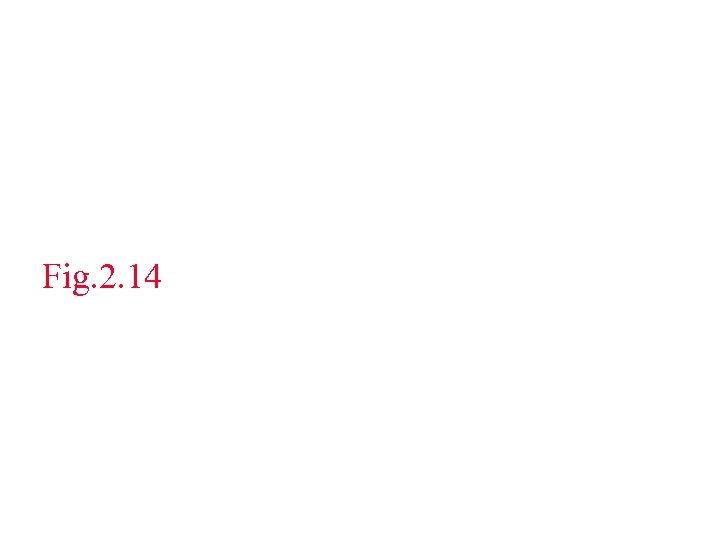Fig. 2. 14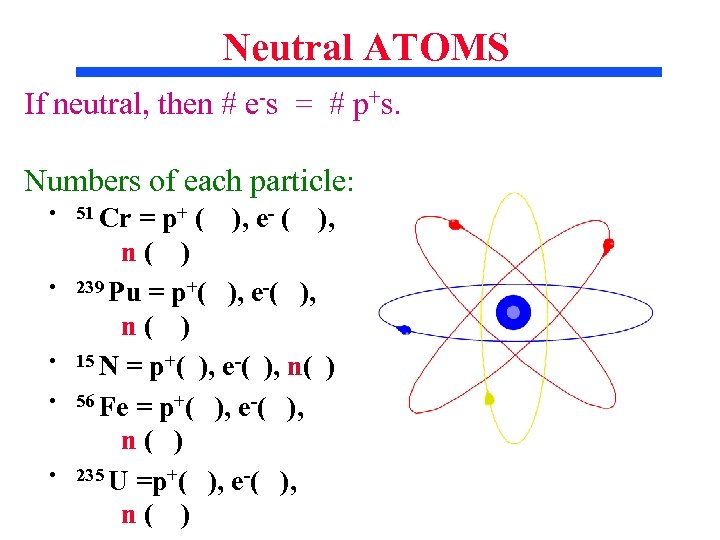Neutral ATOMS If neutral, then # e-s = # p+s. Numbers of each particle: • • • 51 Cr = p+ ( ), e- ( ), n( ) 239 Pu = p+( ), e-( ), n( ) 15 N = p+( ), e-( ), n( ) 56 Fe = p+( ), e-( ), n( ) 235 U =p+( ), e-( ), n( )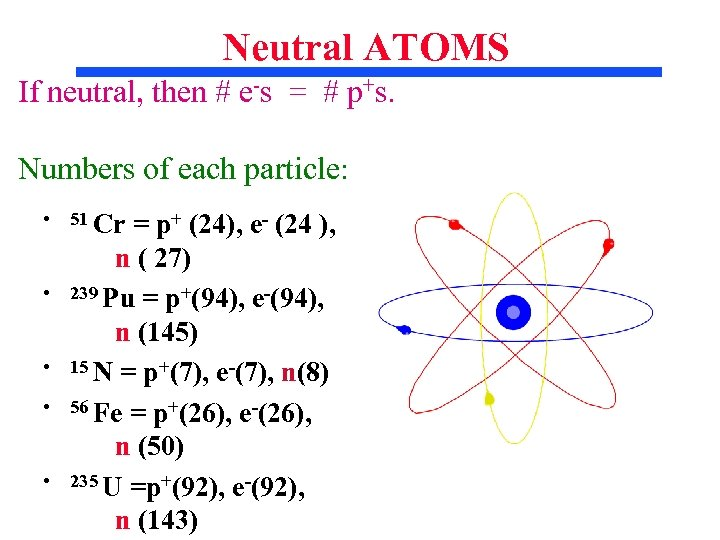Neutral ATOMS If neutral, then # e-s = # p+s. Numbers of each particle: • • • 51 Cr = p+ (24), e- (24 ), n ( 27) 239 Pu = p+(94), e-(94), n (145) 15 N = p+(7), e-(7), n(8) 56 Fe = p+(26), e-(26), n (50) 235 U =p+(92), e-(92), n (143)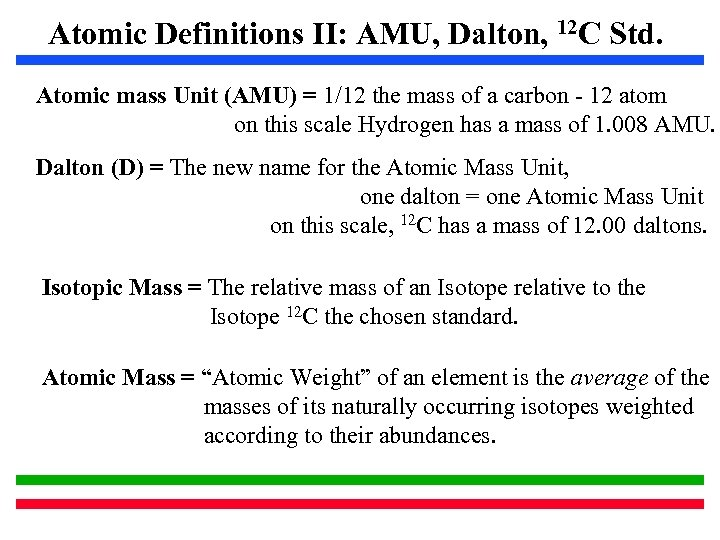Atomic Definitions II: AMU, Dalton, 12 C Std. Atomic mass Unit (AMU) = 1/12 the mass of a carbon - 12 atom on this scale Hydrogen has a mass of 1. 008 AMU. Dalton (D) = The new name for the Atomic Mass Unit, one dalton = one Atomic Mass Unit on this scale, 12 C has a mass of 12. 00 daltons. Isotopic Mass = The relative mass of an Isotope relative to the Isotope 12 C the chosen standard. Atomic Mass = “Atomic Weight” of an element is the average of the masses of its naturally occurring isotopes weighted according to their abundances.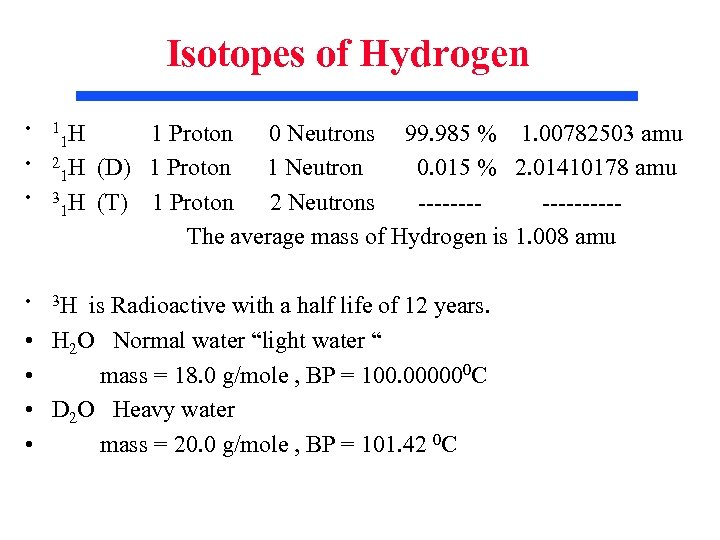Isotopes of Hydrogen • • 1 H 1 2 H 1 3 H 1 Proton 0 Neutrons 99. 985 % 1. 00782503 amu (D) 1 Proton 1 Neutron 0. 015 % 2. 01410178 amu (T) 1 Proton 2 Neutrons --------The average mass of Hydrogen is 1. 008 amu is Radioactive with a half life of 12 years. H 2 O Normal water “light water “ mass = 18. 0 g/mole , BP = 100. 000000 C D 2 O Heavy water mass = 20. 0 g/mole , BP = 101. 42 0 C